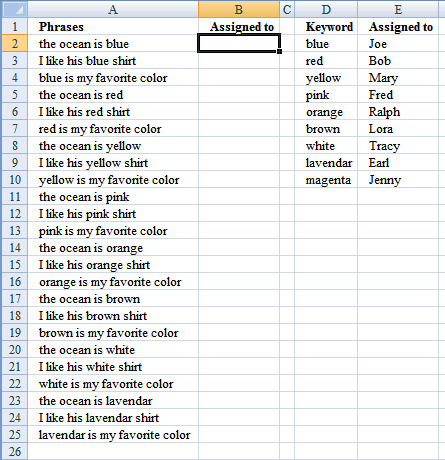Author: Oscar Cronquist Article last updated on January 07, 2019Mr.Excel had a "challenge of the month" June/July 2008 about Wildcard VLOOKUP:

"The data in column A contains a series of phrases. Every phrase contains one color in the phrase. The goal of the challenge is to use the lookup table in D2:E10 to assign the phrase to one of the names in column E."
Source: http://www.mrexcel.com/pc18.shtml

The winner created this formula to return last match in the range:

=LOOKUP(2^15,SEARCH(D\$2:D\$10,A2),E\$2:E\$10)

The third winner used circular reference and iterations to return multiple matches.

### How to return multiple matches without circular referencesArray formula in cell C2:

=INDEX(\$G\$2:\$G\$12, SMALL(IF(COUNTIF(\$A2,"*"&\$D\$2:\$D\$12&"*"), ROW(\$D\$1:\$D\$12), ""), COLUMN(A1)))

Recommended article:

Search for a text string and return multiple adjacent values

This article demonstrates array formulas that search for cell values containing a search string and returns corresponding values on the […]

Alternative array formula in C2:

=INDEX(\$G\$2:\$G\$12, SMALL(IF(ISNUMBER(SEARCH(" "&\$D\$2:\$D\$12&" ", " "&\$A2&" ")), ROW(\$D\$2:\$D\$12)-MIN(ROW(\$D\$2:\$D\$12))+1, ""), COLUMN(A1)))

How to create an array formula

1. Select cell C2
2. Copy above array formula
3. Paste to formula bar
4. Press and hold Ctrl + Shift
5. Press Enter once
6. Release all keys

Copy cell C2 and paste it right as far as needed. Then copy cells and paste down as far as needed.

How to copy array formula

1. Select cell C2
2. Copy (Ctrl + c)
3. Select cell D2
4. Paste (Ctrl + v)

### Explaining array formula in cell C2

=INDEX(\$G\$2:\$G\$12, SMALL(IF(COUNTIF(\$A2,"*"&\$F\$2:\$F\$12&"*"), ROW(\$F\$1:\$F\$11), ""), COLUMN(A1)))

Step 1 - Find text string

The COUNTIF function counts values based on a condition or criteria, in this case two asterisks are appended to the second argument. This makes the COUNTIF function count any value that contains the string, asterisk matches zero or more characters.

COUNTIF(\$A2,"*"&\$F\$2:\$F\$12&"*")

becomes

COUNTIF("the ocean is blue",{"*blue*"; "*red*"; "*yellow*"; "*pink*"; "*orange*"; "*brown*"; "*white*"; "*lavendar*"; "*magenta*"; "*ocean*"; "*shirt*"})

and returns {1; 0; 0; 0; 0; 0; 0; 0; 0; 1; 0}

Step 2 - Convert array to row numbers

The IF function has three arguments, the first one must be a logical expression. If the expression evaluates to TRUE then one thing happens (argument 2) and if FALSE another thing happens (argument 3).

IF(COUNTIF(\$A2,"*"&\$F\$2:\$F\$12&"*"), ROW(\$F\$1:\$F\$11), "")

becomes

IF({1; 0; 0; 0; 0; 0; 0; 0; 0; 1; 0}, {1; 2; 3; 4; 5; 6; 7; 8; 9; 10; 11}, "")

and returns {1; ""; ""; ""; ""; ""; ""; ""; ""; 10; ""}

Step 3 - Return the k-th smallest row number in this data set

To be able to return a new value in a cell each I use the SMALL function to filter column numbers from smallest to largest. SMALL( array, k) The second argument changes when the cell is copied to cells below, this will extract a new value in each cell.

SMALL(IF(COUNTIF(\$A2,"*"&\$F\$2:\$F\$12&"*"), ROW(\$F\$1:\$F\$11), ""), COLUMN(A1))

becomes

SMALL({1; ""; ""; ""; ""; ""; ""; ""; ""; 10; ""},1)

and returns 1.

Step 4 - Return a value or reference of the cell at the intersection of a particular row and column

The INDEX function returns a value based on a cell reference and column/row numbers.

INDEX(\$G\$2:\$G\$12, SMALL(IF(COUNTIF(\$A2,"*"&\$F\$2:\$F\$12&"*"), ROW(\$F\$1:\$F\$11), ""), COLUMN(A1)))

becomes

=INDEX(\$G\$2:\$G\$12, 1)

becomes

=INDEX({"Joe"; "Bob"; "Mary"; "Fred"; "Ralph"; "Lora"; "Tracy"; "Earl"; "Jenny"; "John"; "Theresa"}, 1)

and returns "Joe" in cell C2.